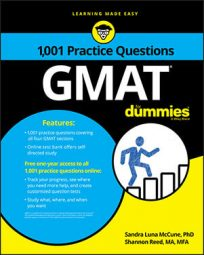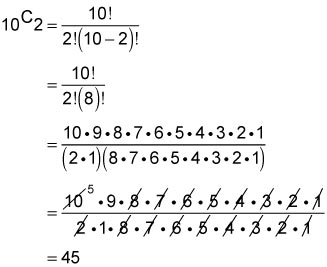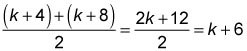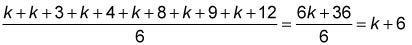##### GMATSome Problem Solving questions in the Quantitative section of the GMAT involve probability and statistics. You should have an understanding of counting techniques, permutations and combinations, basic probability, arithmetic mean, median, mode, and standard deviation.

Problem Solving questions are multiple-choice questions with five answer choice options. You must select the one best answer choice.

## Practice questions

1. In a certain youth soccer league, there are 10 teams. Each game in the season is a match between two teams from the league. If each team plays each of the other teams exactly one time, how many games are played in a season?

A. 20 B. 50 C. 45 D. 90 E. 100

2. What is the difference between the mean and the median of the positive numbers, k, k + 3, k + 4, k + 8, k + 9, k + 12?

A. 0 B. 1 C. 6 D. 2k E. 5k

1. The correct answer is C.

This is a combination counting question. You can answer the question by finding the combination of 10 items (teams) taken 2 at a time. Using the combination formula, you haveThus, there are 45 games in the season. (Note: Recall that n! (read "n factorial") is the product of all positive integers descending down from n to 1.)

2. The correct answer is A.

The six positive numbers are in ascending order, so the median is the average of the middle pair of numbers, which isThe mean is the sum of the numbers divided by 6, which isThe mean and the median both equal k + 6, so the difference is 0.